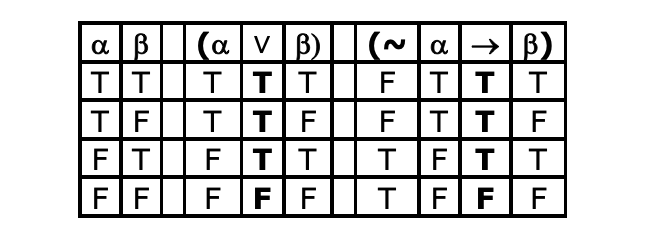## Want to keep learning?

This content is taken from the University of York's online course, Logic: The Language of Truth. Join the course to learn more.
4.6

## University of York# Arrow and ‘if … then … ’: an argument for equivalence

Here is an argument for the view that arrow and ‘if … then … ’ have the same truth-table. This argument uses some of the key resources we’ve developed in this course.

First, note that where ‘If α then β’ is true, that’s enough to guarantee that ‘(α $$\rightarrow$$ β)’ is true: we’ve already noted that where ‘If α then β’ is true, it can’t be the case that ‘α’ is true and ‘β’ is false, and that’s the only kind of situation in which‘(α $$\rightarrow$$ β)’ can be false. So, ‘If α then β’ entails ‘(α $$\rightarrow$$ β)’.

Now, look at this simple argument:

• Boris did it or Dominic did it
• Therefore, if it’s not the case that Boris did it, then Dominic did it

Note that this argument is expressed in English and uses ‘If … then … ’. It seems that it is valid. More than that, it seems to be formally valid. Any argument with this shape

• α or β
• Therefore, if ~α, then β

will be valid.

But now notice that ‘or’ and vel have the same truth-table. So, we can substitute vel for ‘or’ in the premise, like this:

• (α $$\vee$$ β)
• Therefore, if ~α, then β

(If you are worried about exclusive-‘or’ here, note that there do seem to be cases of ‘or’ in English which are inclusive, and someone could plausibly say the premise of our example argument intending inclusive-‘or’ and the argument would be valid.)

But now look at the truth-tables for ‘(α $$\vee$$ β)’ and ‘(~α $$\rightarrow$$ β)’.#### Figure 1. Truth-table for ‘(α $$\vee$$ β)’ and ‘(~α $$\rightarrow$$ β)’

What does this show about ‘(α $$\vee$$ β)’ and ‘(~α $$\rightarrow$$ β)’?

It shows they are logically equivalent. So, as far as valid arguments go, we can swap one for the other and, if our original argument was valid, the argument resulting from the substitution will be valid too.

Making the substitution gives us this:

• (~α $$\rightarrow$$ β)
• Therefore, if ~α, then β

But now look what we’ve got. We started with an argument in English that we are confident is formally valid. We’ve substituted logically equivalent sentences for the original premise, but the result is that we have an argument (a formally valid argument) from an arrow-sentence to the corresponding ‘If … then … ’-sentence. Putting our two results together, we have:

• If α then β $$\models$$ (α $$\rightarrow$$ β)

and

• (α $$\rightarrow$$ β) $$\models$$ If α then β

This means ‘If α then β’ and ‘(α $$\rightarrow$$ β)’ are logically equivalent. Arrow and ‘If … then … ’ do have the same truth-table.